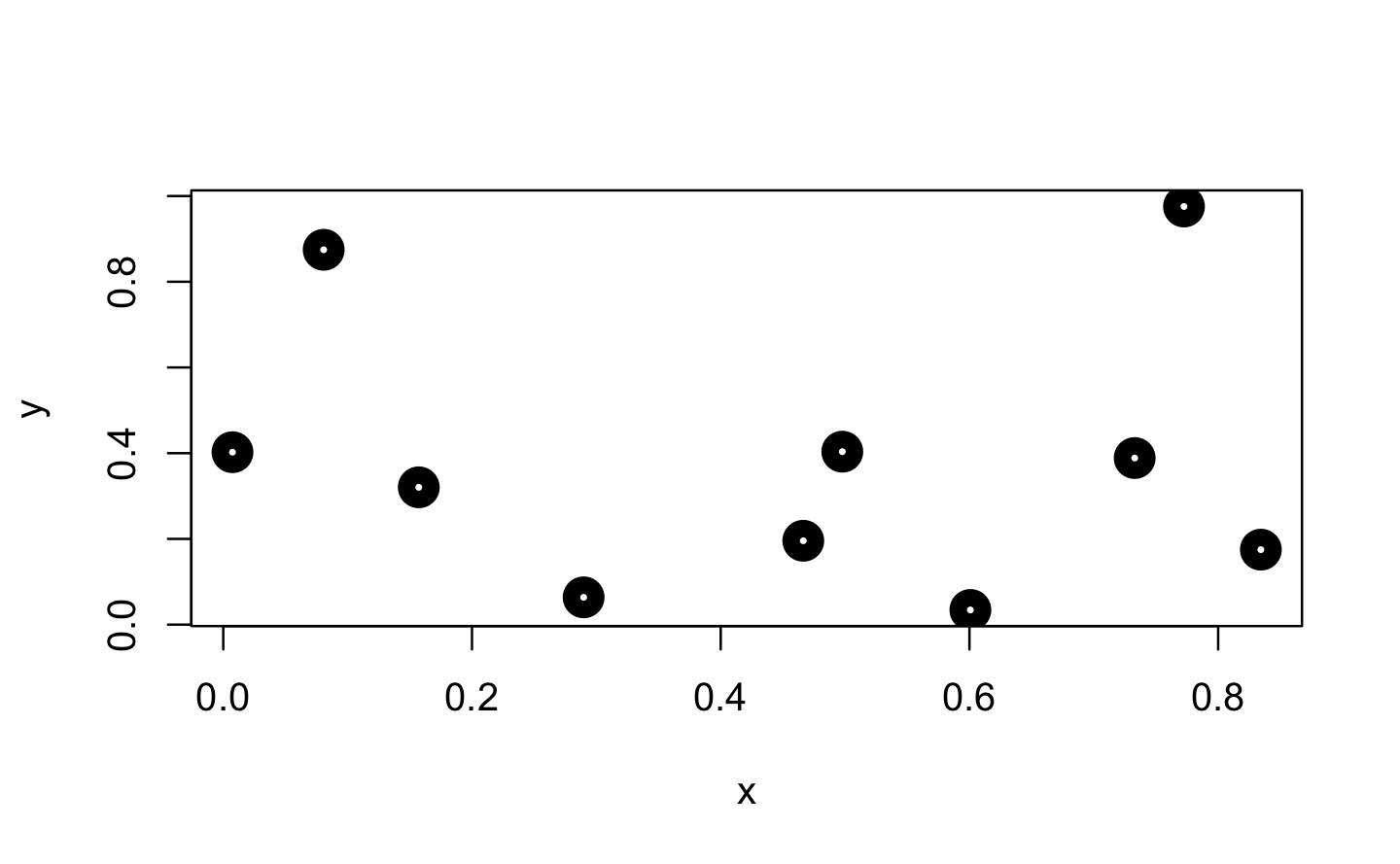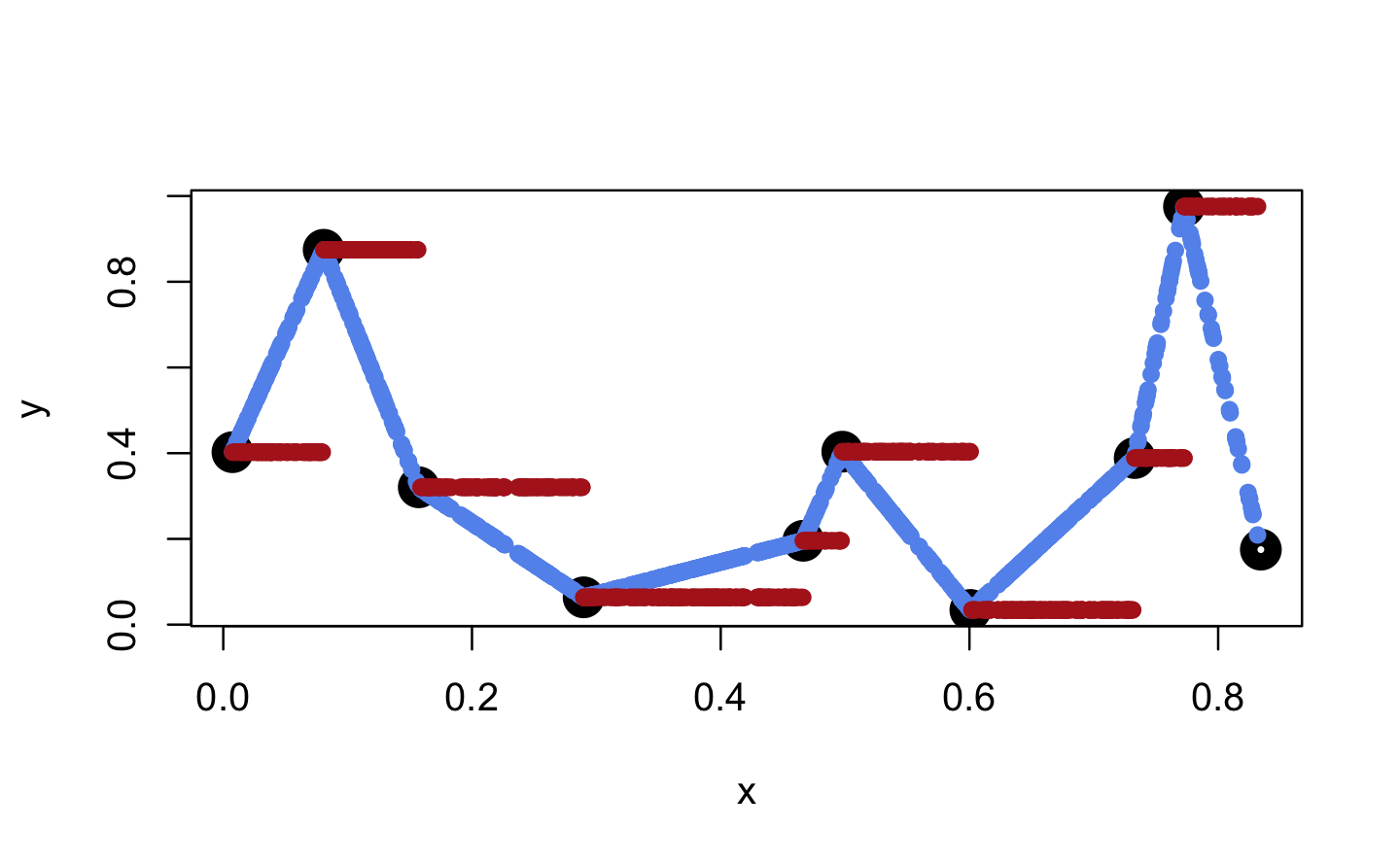# 9 Function factories

## Prerequisites

For most of this chapter base R15 is sufficient. Just a few exercises require the rlang,16 dplyr,17 purrr18 and ggplot219 packages.

library(rlang)
library(dplyr)
library(purrr)
library(ggplot2)

## 9.1 Factory fundamentals

Q1: The definition of force() is simple:

force
#> function (x)
#> x
#> <bytecode: 0x7fe0e09464b0>
#> <environment: namespace:base>

Why is it better to force(x) instead of just x?

A: As you can see force(x) is similar to x. As mentioned in Advanced R, we prefer this explicit form, because

using this function clearly indicates that you’re forcing evaluation, not that you’ve accidentally typed x."

Q2: Base R contains two function factories, approxfun() and ecdf(). Read their documentation and experiment to figure out what the functions do and what they return.

A: Let’s begin with approxfun() as it is used within ecdf() as well:

approxfun() takes a combination of data points (x and y values) as input and returns a stepwise linear (or constant) interpolation function. To find out what this means exactly, we first create a few random data points.

x <- runif(10)
y <- runif(10)
plot(x, y, lwd = 10)Next, we use approxfun() to construct the linear and constant interpolation functions for our x and y values.

f_lin <- approxfun(x, y)
f_con <- approxfun(x, y, method = "constant")

# Both functions exactly reproduce their input y values
identical(f_lin(x), y)
#>  TRUE
identical(f_con(x), y)
#>  TRUE

When we apply these functions to new x values, these are mapped to the lines connecting the initial y values (linear case) or to the same y value as for the next smallest initial x value (constant case).

x_new <- runif(1000)

plot(x, y, lwd = 10)
points(x_new, f_lin(x_new), col = "cornflowerblue", pch = 16)
points(x_new, f_con(x_new), col = "firebrick", pch = 16)However, both functions are only defined within range(x).

f_lin(range(x))
#>  0.402 0.175
f_con(range(x))
#>  0.402 0.175

median   = performances$median ) ggplot(df_perf, aes(x_length, median, col = method)) + geom_point(size = 2) + geom_line(linetype = 2) + scale_x_log10() + labs( x = "Length of x", y = "Execution Time (ms)", color = "Method" ) + theme(legend.position = "top")## 9.4 Function factories + functionals Q1: Which of the following commands is equivalent to with(x, f(z))? 1. x$f(x$z). 2. f(x$z).
3. x$f(z). 4. f(z). 5. It depends. A: (e) “It depends” is the correct answer. Usually with() is used with a data frame, so you’d usually expect (b), but if x is a list, it could be any of the options. f <- mean z <- 1 x <- list(f = mean, z = 1) identical(with(x, f(z)), x$f(x$z)) #>  TRUE identical(with(x, f(z)), f(x$z))
#>  TRUE
identical(with(x, f(z)), x\$f(z))
#>  TRUE
identical(with(x, f(z)), f(z))
#>  TRUE

Q2: Compare and contrast the effects of env_bind() vs. attach() for the following code.

funs <- list(
mean = function(x) mean(x, na.rm = TRUE),
sum = function(x) sum(x, na.rm = TRUE)
)

attach(funs)
#> The following objects are masked from package:base:
#>
#>     mean, sum
mean <- function(x) stop("Hi!")
detach(funs)

env_bind(globalenv(), !!!funs)
mean <- function(x) stop("Hi!")
env_unbind(globalenv(), names(funs))

A: attach() adds funs to the search path. Therefore, the provided functions are found before their respective versions from the {base} package. Further, they cannot get accidentally overwritten by similar named functions in the global environment. One annoying downside of using attach() is the possibility to attach the same object multiple times, making it necessary to call detach() equally often.

attach(funs)
#> The following objects are masked from package:base:
#>
#>     mean, sum
attach(funs)
#> The following objects are masked from funs (pos = 3):
#>
#>     mean, sum
#>
#> The following objects are masked from package:base:
#>
#>     mean, sum

detach(funs)
In contrast rlang::env_bind() just adds the functions in fun to the global environment. No further side effects are introduced, and the functions are overwritten when similarly named functions are defined.
env_bind(globalenv(), !!!funs)
#>  "package:rlang"   "package:stats"